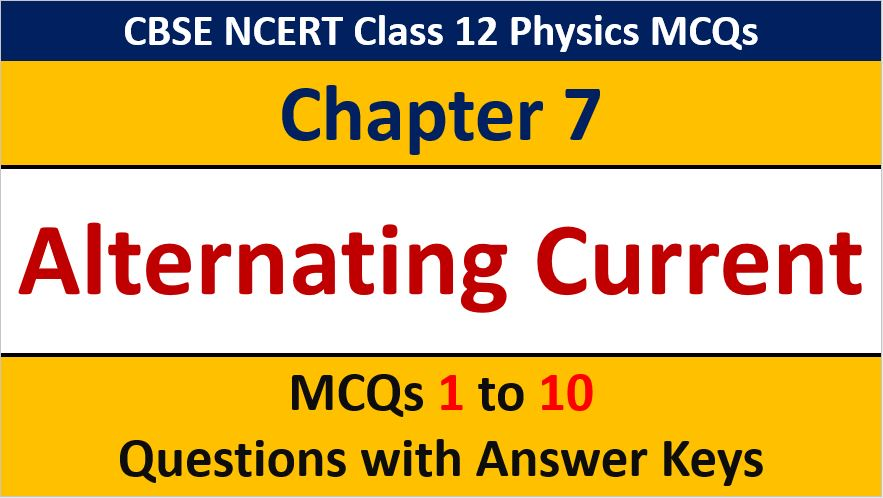## MCQ Questions for Class 12 Physics Chapter 7 Alternating Current with Answer Keys

If you are preparing for CBSE Class 12 Board Exam Term 1 which will be an objective type questions paper, then you need to practice multiple choice questions of Class 12 Physics Chapterwise. In this article of AKVTutorials, you will get MCQ Questions for Class 12 Physics Chapter 7 Alternating Current with Answer Keys.

MCQ Questions for Class 12 Physics Chapter 7 Question No 1:

Alternating current is transmitted to distant places at

Option A : High voltage and low current

Option B : High voltage and high current

Option C : Low voltage and low current

Option D : Low voltage and high current

Option A : High voltage and low current

MCQ Questions for Class 12 Physics Chapter 7 Question No 2:

The average e.m.f duration the positive half cycle of an AC supply of peak value Eis

Option A : E/ π

Option B : E/ √2

Option C : E/ √2 π

Option D : 2 E/ π

Option D : 2 E/ π

MCQ Questions for Class 12 Physics Chapter 7 Question No 3:

The correct graph between the resistance of a conductor with frequency is

Option A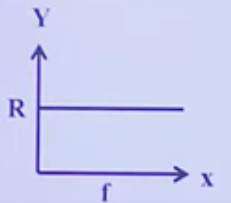Option B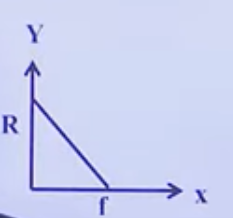Option C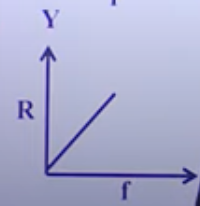Option D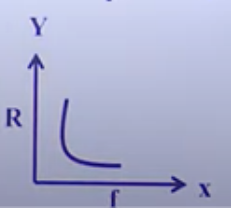Option A :

MCQ Questions for Class 12 Physics Chapter 7 Question No 4:

Voltages across R,L,C are V, V, V3 respectively. Then the voltage of applied AC source is always equal to

Option A : V1 + V2 + V3

Option B : √ V1 2+ (V2 + V3)2

Option C : V1 – V2 – V3

Option D : √ V1 2+ (V2 – V3)2

Option D : √ V1 2+ (V2 – V3)2

MCQ Questions for Class 12 Physics Chapter 7 Question No 5:

In series L-C-R resonant circuit, to increase the resonant frequency

option A : L will have to be increased

Option B : C will have to be increased

Option C : LC will have to be decreased

Option D : None

Option C : LC will have to be decreased

MCQ Questions for Class 12 Physics Chapter 7 Question No 6:

In the circuit shown in the figure, neglecting source resistance, the voltmeter and ammeter readings will respectively be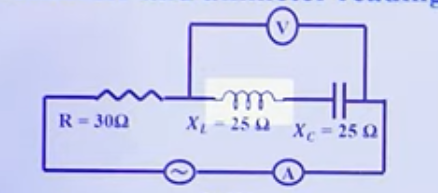Option A : 0V, 8 A

Option B : 150 V, 8V

Option C : 150 V, 3V

Option D : 0.V, 3A

Option A : 0V, 8 A

MCQ Questions for Class 12 Physics Chapter 7 Question No 7:

The r.m.s. value of an ac of 50Hz is 10amp. The time taken by the alternating current in reaching from zero to maximum value and the peak value of current will be

Option A : 2 × 10-2 sec and 14.14 amp

Option B : 1 × 10-2 sec and 7.07 amp

Option C : 5 × 10-3 sec and 7.07 amp

Option D : 5 × 10-3 sec and 14.14 amp

Option D : 5 × 10-3 sec and 14.14 amp

MCQ Questions for Class 12 Physics Chapter 7 Question No 8:

The magnitude of the emf across the secondary of a transformer does not depend on

Option A : The number of the turns in the primary

Option B : The number of the turns in the secondary

Option C : The magnetic of the emf applied across the primary

Option D : The resistance of the primary and the secondary

Option D : The resistance of the primary and the secondary

MCQ Questions for Class 12 Physics Chapter 7 Question No 9:

In case of ac circuit, Ohm’s law holds good for

Option A : Peak values of voltage and current

Option B : Effective value of voltage and current

Option C : Instantaneous values of voltage and current

Option D : All the above

Option D :All the above

MCQ Questions for Class 12 Physics Chapter 1 Question No 10:

In case of AC circuits the relation V = i Z, where Z is impedance, can be directly applied to

Option A : Peak values of voltage and current only

Option B : Runs values of voltage and current only

Option C : Instantaneous values of voltage and current only

Option D : Both 1 and 2 are true

Option D : Both 1 and 2 are true

CBSE Class 12 Physics Chapterwise MCQ
1 : Electric Charge & Fields MCQs
| 1 to 10 | 11 to 20
2 : Electrostatics Potential and Capacitance MCQs
| 1 to 10 | 11 to 20
3 : Current Electricity MCQs
| 1 to 10 | 11 to 20
4 : Moving charges and Magnetism MCQs
| 1 to 10 | 11 to 20
5 : Magnetism and Matter MCQs
| 1 to 10 | 11 to 20
6 : Electromagnetic Induction MCQs
| 1 to 10 | 11 to 20
7 : Alternating Current MCQs
| 1 to 10 | 11 to 20
8 : Electromagnetic Waves MCQs
| 1 to 10 | 11 to 20
9 : Ray Optics and Optical Instruments MCQs
| 1 to 10 | 11 to 20
10 : Wave Optics MCQs
| 1 to 10 | 11 to 20
11 : Dual Nature of Radiation MCQs
| 1 to 10 | 11 to 20
12 : Atoms MCQs
| 1 to 10 | 11 to 20
13 : Nuclei MCQs
| 1 to 10 | 11 to 20
14 : Semi Conductor Electronics MCQs
| 1 to 10 | 11 to 20
15 : Communication Systems MCQs
| 1 to 10 | 11 to 20